30 60 90 Triangles homework Help at TutorEye

# Best Homework Help For 30 60 90 Triangles

## Top Questions

t schedule 3 people for the day my % scheduled is 78.57% My question is how did the computer come up with the 78.57%?
View More

I would love to get a written solution. I did the other proof tree that you can see in the attaached files. So its supposed to look like that Thank You.
View More

xtbook. i really need in in half an hour
View More

## 30 60 90 Triangles:

The 30-60-90 triangle is a special right triangle, as it has a special relationship between its sides. If we know the measure of at least one side of the triangle, the special proportions of sides of the 30-60-90 triangle could be used to determine the measure of other sides of the same triangle.

These special relations between the sides of the 30-60-90 triangle are the result of the Pythagorean theorem.## 30 60 90 Triangles Sample Questions:

Question 1: In the following 30-60-90 triangle, determine the measure of the hypotenuse.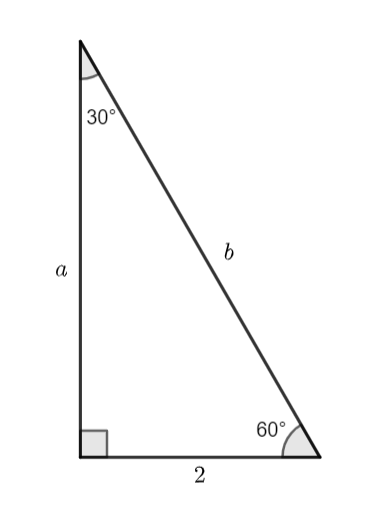Explanation: The given measure is the shorter leg of the 30-60-90 triangle. And, we know the hypotenuse of the 30-60-90 triangle, is twice the measure of the shorter leg.

Question 2: In the following 30-60-90 triangle, determine the measure of the longer side.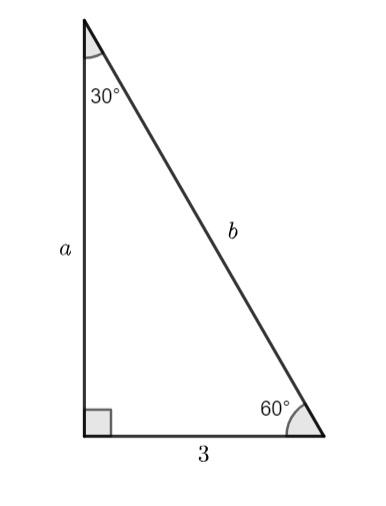Explanation: The given measure is the shorter leg of the 30-60-90 triangle. And, we know the longer side of the 30-60-90 triangle, is square root three times the measure of the shorter leg.

Question 3: Determine the measure of the shorter side.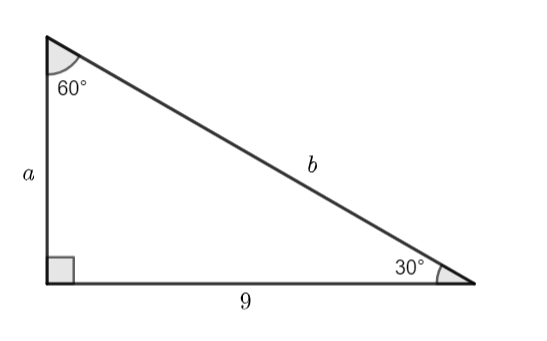Explanation: The given measure is the longer leg of the 30-60-90 triangle. And, we know the longer side of the 30-60-90 triangle, is square root three times the measure of the shorter leg.

Question 4: Determine the measure of the hypotenuse.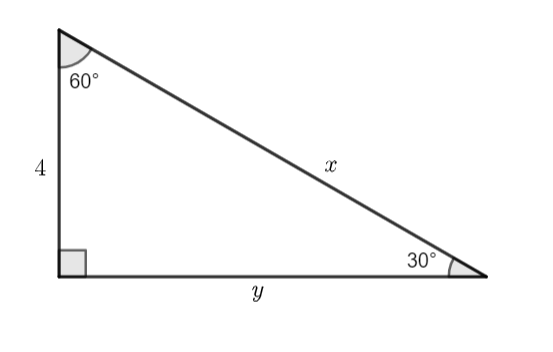Explanation: The given measure is the shorter leg of the triangle. And, we know the hypotenuse of the 30-60-90 triangle, is twice the measure of the shorter leg.

Question 5: Does the length of sides 2 cm, 23 cm, and 4 cm, represent a 30-60-90 triangle?

Explanation: The given measure of the sides are: 2 cm, 2√3 cm, and 4 cm.

Now, let us divide the proportion by 2. We get, 1 cm, √3 cm, and 2 cm.

Question 6: Does the length of sides 3 cm, 63 cm, and 9 cm, represent a 30-60-90 triangle?

Explanation: The given measure of the sides are: 3 cm, 6√3 cm, and 9 cm.

Now, let us divide the proportion by 3. We get, 1 cm, 2√3 cm, and 2 cm.

Question 7: For the following 30-60-90 triangle, determine the measure of the unknown side.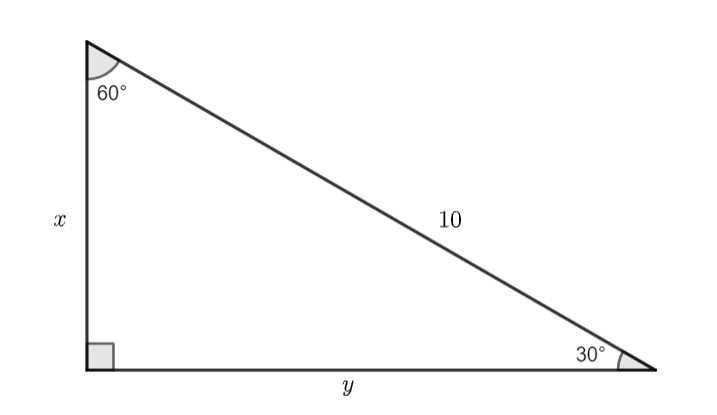Explanation: We have given the measure of the hypotenuse. And, we know the hypotenuse of the 30-60-90 triangle, is twice the measure of the shorter leg.

Question 8: Determine the measure of the unknown side.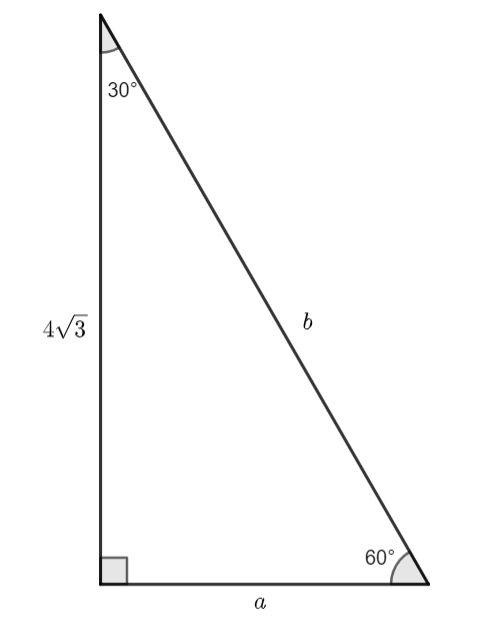Explanation: We have given the measure of the longer side. And, we know the longer side of the 30-60-90 triangle is square root three times the measure of the shorter leg.

Question 9: The length of the hypotenuse of the 30-60-90 triangle is 12 cm. What would be the measure of the other two sides?

Explanation: We have given the measure of the hypotenuse. And, we know the hypotenuse of the 30-60-90 triangle, is twice the measure of the shorter leg.

Question 10: The length of the longer side of the 30-60-90 triangle is 73 cm. What would be the measure of the other two sides?

Explanation: We have given the measure of the longer side. And, we know the longer side of the 30-60-90 triangle is square root three times the measure of the shorter leg.

Question 1: How to solve 30 60 90 triangles?

30-60-90 is a special type of right triangle in which angles are in the ratio of 1:2:3 and sides are in ratio 1:√3:2.

We can solve according to the given values of the corresponding sides.Question 2: How to find the sides of a 30 60 90 triangle?

In 30-60-90 triangles we use the triangle rule to find the sides.

• When base BC base is given, by triangle rule we   calculate all the rest sides.• When perpendicular AB is given,by triangle rule we calculate all the rest sides.• When hypotenuse AC is given,by triangle rule we calculate the remaining sidesQuestion 3: Which triangle is a 30°-60°-90° triangle?

A right triangle having angles of  30°,  60°, and 90° is known as a 30-60-90 triangle The sides are always in the same ratio to each other since the angles are always in that ratio.

Question 4: How to find the area of a 30 60 90 triangle?

The total space occupied by the three sides of a triangle is called its area. The basic formula is half of the product of its base and height. The 30-60-90 triangle is a right-angled triangle and its sides are always in the same ratio 1: √3:2.